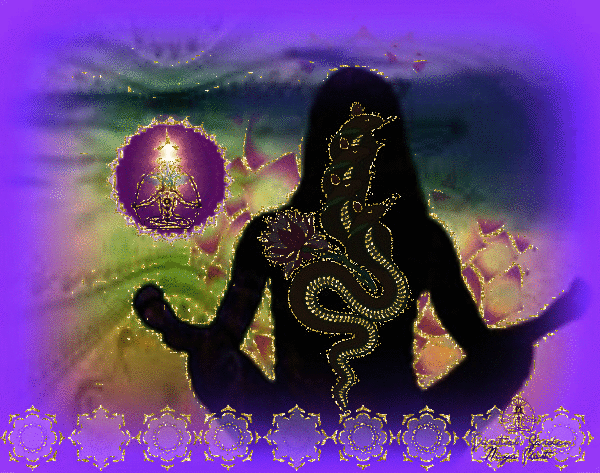you can find yourself over and over again."

Joseph CampbellS h i m m y  S h a m a n  S C H E D U L E

C  O  M  I  N  G    S   O   O   N

S H I M M Y  S H A M A N  Y O G A   w o r k s h o p s    i n c l u d e :

ritual, meditation, nourishing, sensual yoga movement/dance, sound healing, stillness, integration, and healing hugs.

P A R T N E R   Y O G A

S E L F - C A R E   Y O G A   R I T U A L S

G R A T I T U D E  M O V E M E N T  C I R C L E S

R A D I C A L  F O R G I V E N E S S  H E A L I N G  C I R C L E S

C U D D L E   P U D D L E   Y O G A   P A R T Y

E M B R A C E  Y O U R   L I G H T  &  S H A D O W   W O R K S H O P